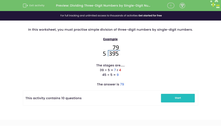# Dividing Three-Digit Numbers by Single-Digit Numbers

In this worksheet, students improve their fluency at dividing three-digit numbers by single-digit numbers.Key stage:  KS 3

Curriculum topic:   Number

Curriculum subtopic:   Use Four Operations for All Numbers

Difficulty level:#### Worksheet Overview

In this worksheet, you must practise simple division of three-digit numbers by single-digit numbers.

ExampleThe stages are.....

39 ÷ 5 = 7 r 4

45 ÷ 5 = 9